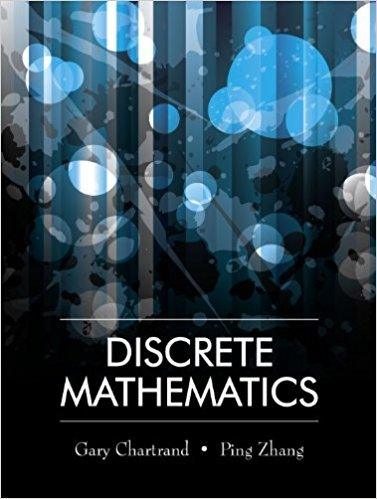×
×

# Solutions for Chapter 8.2: The Principle of Inclusion-Exclusion## Full solutions for Discrete Mathematics | 1st Edition

ISBN: 9781577667308Solutions for Chapter 8.2: The Principle of Inclusion-Exclusion

Solutions for Chapter 8.2
4 5 0 360 Reviews
28
0
##### ISBN: 9781577667308

Chapter 8.2: The Principle of Inclusion-Exclusion includes 17 full step-by-step solutions. Since 17 problems in chapter 8.2: The Principle of Inclusion-Exclusion have been answered, more than 12271 students have viewed full step-by-step solutions from this chapter. Discrete Mathematics was written by and is associated to the ISBN: 9781577667308. This textbook survival guide was created for the textbook: Discrete Mathematics, edition: 1. This expansive textbook survival guide covers the following chapters and their solutions.

Key Math Terms and definitions covered in this textbook
• Big formula for n by n determinants.

Det(A) is a sum of n! terms. For each term: Multiply one entry from each row and column of A: rows in order 1, ... , nand column order given by a permutation P. Each of the n! P 's has a + or - sign.

• Characteristic equation det(A - AI) = O.

The n roots are the eigenvalues of A.

• Cholesky factorization

A = CTC = (L.J]))(L.J]))T for positive definite A.

• Elimination.

A sequence of row operations that reduces A to an upper triangular U or to the reduced form R = rref(A). Then A = LU with multipliers eO in L, or P A = L U with row exchanges in P, or E A = R with an invertible E.

• Factorization

A = L U. If elimination takes A to U without row exchanges, then the lower triangular L with multipliers eij (and eii = 1) brings U back to A.

• Graph G.

Set of n nodes connected pairwise by m edges. A complete graph has all n(n - 1)/2 edges between nodes. A tree has only n - 1 edges and no closed loops.

• Hankel matrix H.

Constant along each antidiagonal; hij depends on i + j.

• Hermitian matrix A H = AT = A.

Complex analog a j i = aU of a symmetric matrix.

• Iterative method.

A sequence of steps intended to approach the desired solution.

• Nullspace matrix N.

The columns of N are the n - r special solutions to As = O.

• Outer product uv T

= column times row = rank one matrix.

• Pivot.

The diagonal entry (first nonzero) at the time when a row is used in elimination.

• Projection p = a(aTblaTa) onto the line through a.

P = aaT laTa has rank l.

• Pseudoinverse A+ (Moore-Penrose inverse).

The n by m matrix that "inverts" A from column space back to row space, with N(A+) = N(AT). A+ A and AA+ are the projection matrices onto the row space and column space. Rank(A +) = rank(A).

• Reduced row echelon form R = rref(A).

Pivots = 1; zeros above and below pivots; the r nonzero rows of R give a basis for the row space of A.

• Row picture of Ax = b.

Each equation gives a plane in Rn; the planes intersect at x.

• Schwarz inequality

Iv·wl < IIvll IIwll.Then IvTAwl2 < (vT Av)(wT Aw) for pos def A.

• Spanning set.

Combinations of VI, ... ,Vm fill the space. The columns of A span C (A)!

• Spectral Theorem A = QAQT.

Real symmetric A has real A'S and orthonormal q's.

• Vandermonde matrix V.

V c = b gives coefficients of p(x) = Co + ... + Cn_IXn- 1 with P(Xi) = bi. Vij = (Xi)j-I and det V = product of (Xk - Xi) for k > i.

×